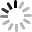# SVG 研究之路 (7) - fill 填色

## 基本純色

``````<rect x="50" y="50" width="100" height="100" stroke="#000" stroke-width="10" fill="#f40"></rect>
<rect x="170" y="50" width="100" height="100" stroke="#000" stroke-width="10" fill="#0f5"></rect>
<rect x="290" y="50" width="100" height="100" stroke="#000" stroke-width="10" fill="#09f"></rect>
``````## 漸層

SVG 裏頭的漸層做法比較特殊，首先要先建立一個漸層的定義檔，接著讓需要漸層的元件，指向這個定義檔，就可以讓元件具有漸層，除了漸層是這麼做的，SVG 文字的變化也有一部分是使用定義檔來表現，會之後的篇幅提到。至於定義檔的寫法，會在外層統一用一個`<defs></defs>`( definitions ) 的標籤包著，這樣也方便識別當中的標籤是定義檔 ( 我測試過不包也是可以，不過基於 W3C 的定義規則，還是包起來吧 )，接著在`<defs></defs>`裏頭加入`<linearGradient></linearGradient>`的標籤，這就完成了線性漸層漸層定義檔的架構 ( 放射狀漸層使用`<radialGradient></radialGradient>` ) ，不過別急，還沒結束，`<linearGradient></linearGradient>`裏頭還有漸層顏色的設定，就是使用`<stop>`的標籤來設定，可以看看下面的設定：

``````<defs>
<stop offset="5%" stop-color="#F00" />
<stop offset="95%" stop-color="#ff0" />
</defs>
``````

``````<rect x="50" y="250" width="100" height="100" stroke="#000" stroke-width="5" fill="url(#a1)"></rect>
<circle cx="220" cy="300" r="50" stroke="#000" stroke-width="5" fill="url(#a1)"></circle>
<rect x="290" y="250" width="100" height="100" stroke="url(#a1)" stroke-width="5" fill="none"></rect>
````````````<defs>
<stop offset="5%" stop-color="#ff0" />
<stop offset="95%" stop-color="#f00" />
</defs>
````````````<defs>
<linearGradient id="a1" x1="0%" y1="0%" x2="0%" y2="100%">
<stop offset="5%" stop-color="#F00" />
<stop offset="95%" stop-color="#ff0" />
</defs>
````````````<defs>
<linearGradient id="a1" x1="0%" y1="0%" x2="100%" y2="100%">
<stop offset="5%" stop-color="#F00" />
<stop offset="95%" stop-color="#ff0" />
</defs>
````````````<defs>
<stop offset="5%" stop-color="#ff0" />
<stop offset="95%" stop-color="#f00" />
</defs>
``````## 圖案

``````<defs>
<pattern id="a2" patternUnits="userSpaceOnUse" x="0" y="0" width="30" height="30">
<rect x="0" y="0" width="25" height="25" stroke="#none" fill="#09f"></rect>
</pattern>
</defs>
``````

``````<circle cx="220" cy="300" r="50" stroke="#000" stroke-width="5" fill="url(#a2)"></circle>
``````